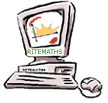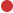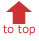Project Home Publications & Seminars Research Materials Project Team OnlyAnnulus Area ProblemCompleting the SquareGolden RatioMarina's fish shopPipe ProblemTransformations of the Graph of a Quadratic FunctionQuadratics With Gridpic and Still ImagesYou might also look at our section on surds

In the following Power Point presentation Professor Kaye Stacey provides an overview of the key topics and themes that make up the study of Quadratic Funtions and Equations in secondary school mathematics.

Quadratic Functions and Equations: An Overview (zipped ppt)

Annulus Area Problem

This problem uses the need for a gardener to find the area of circular garden path surrounding a circular pool as its real-life context. This can initiate an exploration of the relationship between the area of an annulus and the length of the chord of the larger circle that is tangent to the inner circle of the annulus. The rule for the quadratic function can be found by use of the Theorem of Pythagoras.

AnnulusAreaProbSheet (pdf) - student sheet with a statement of the problem and a diagram

AnnulusAreaGSPSketch (zipped gsp) - a Geometer's Sketchpad sketch with a page giving the problem and a diagram that can be changed, a second page for collecting and plotting data from the diagram, a third page for exploring the functional relationship between variables in the problem, and a final page showing the function rule; link buttons can be used to move through the pages in sequence.

Area of Equilateral Triangle, Square and Circle for Fixed Perimeter

The question: - For a perimeter of fixed length, which shape, a triangle, a square or a circle, will enclose the largest area? - can provide the stimulus for this exploration. This leads to finding out which type of triangle encloses the largest area and then to finding rules for the areas of the three shapes for a fixed perimeter. These rules are all quadratic functions of the length of the fixed perimeter, and can be found using known formulae for area and perimeter of the shapes, along with the Theorem of Pythagoras for the triangle.

TriangleMaxAreaFixedPerimeterGSPSketch (zipped gsp) - A Geometer's Sketchpad sketch that allows the lengths of the sides of a triangle to be changed to first show that when the length of one side is fixed, the other two sides need to be of the same length to give the largest area; and then when the length of the first side is changed to give the largest area when the lengths of the other two sides are the same the result is an equilateral triangle

MaxAreaTriSqCircleFixedPerimeterSketch (zipped gsp) - A Geometer's Sketchpad sketch that graphically shows the area of an equilateral triangle, a square and a circle as a function of its perimeter; enabling comparisons to be made to determine which shape gives the largest area for a given fixed perimeter, and motivating the determination of the function rules for the areas.

Video clips of BMX bicycle stunt riders in action provide the context for engaging students in quadratic modeling of paths and analysing quadratic functions. The video analysis tool Vidshell (See Maths from Still and Moving Images) is used in the investigation of the paths of the riders as seen in the movie clips. Graphics calclulators can be used to fit a least-squares quadratic to data sets obtained for these paths.

Slide Show(zipped ppt) - Power Point show used by B. McCann and R. Pierce in their presentation of BMX Bicycles, Dirt Jumps, Movies and Mathematics at the 42nd Annual Conference of the Mathematical Association of Victoria in December, 2005.

BMX Bikes & Quadratics Lesson Sheets - a zipped file that contains the following three items:

Lesson Plan (pdf) - a lesson plan for the teacher

Activity Sheet (pdf) - student sheet giving instructions and questions to be answered by students

Instruction Sheet (pdf) - sheet with BMX video clip and Vidshell instructions, and also graphics calculator commands for finding the least squares regression line from data in lists

Movies - two digital movie clips, BenDirtJump and AhmedDirtJump, to be used together with VidShell to collect data linking the vertical height of the rider with the horizontal distance travelled over the path of the rider's jumpBenDirtJump (zipped)AhmedDirtJump (zipped)

Vidshell Software - highly recommended free software created by Doyle V. Davis for collecting and analysing data from movies; fairly easy to use, has a good range of basic capabilities, and comes with a rich supply of good videos to work on

Completing the Square Diagram

New life is given to a static diagram from an old problem found in the work of Mohammad al-Khwarizmi. The new dynamic diagram visually shows in a colourful and engaging way the use of his procedure (now known as "Completing the Square") for solving a quadratic equation.

CompleteSquareGSPSketch (zipped gsp) - a presentation diagram with sequenced steps showing the use of completing the square to find the solution of a quadratic equation to solve a problem posed and solved with this method by al-Khwarizmi around 820 A.D.

Danish Flag Problem

The Danish Flag, with its white cross with a red background, provides the context for this problem. Students are asked to find the width of the white cross so that its area is linked in a specified way to the area of red on the flag.

DanishFlagGuided (pdf) - student sheet providing instructions and questions to be answered by students where some guidance is provided to the student

DanishFlagNotGuided (pdf) - student sheet providing instructions and questions to be answered by students without trying to lead students to see how they should answer the questions

DanishFlagGSPSketch (zipped gsp) - a Geometer's Sketchpad sketch that enables the students to explore the funtional relationships for the area of white and area of red on the flag, approximate when the two areas are equal by finding when their graphs intersect, and link this result to finding the solution of a quadratic equation resulting from equating the two area functions.

Golden Ratio

The golden ratio is introduced in the real-context of ratio of distances related to eyes. From this students move on to linking the golden ratio to the solution of a quadratic equation.

GoldenRatioSlideShow (ppt) - a Power Point Show that introduces the Golden Ratio from ratios of distances related to eyes, asks student to find and solve a quadratic equation that gives the raio and show some other real life situations involving the Golden Ratio

GoldenRatioEyesGSPSketch (zipped gsp) - a Geometer's Sketchpad Sketch where eye distances are changed to introduce the Golden Ratio as the number when two special ratios are equal

GraphsEyeRatios (zipped gsp) - a Geometer's Sketchpad Sketch where the ratios linked to eye distances are graphed to find when they are equal

GoldenRatioWorksheet (pdf) - a student worksheet that asks students to find a quadratic based on the ratio of line segments that has as a solution the Golden Ration value

QuadraticFromEyeRatios (gsp) - a Geometer's Sketchpad Sketch where the ratios linked to eye distances are used to find a quadratic equation that has as a solution the Golden Ratio value

GoldenRatioAll - zipped file containing all of the individual files above.

There is more material on the golden ratio on the page about surds.

Marina's fish shop

In this 100-minute lesson (or 2×50 minutes)  for Year 10 students, the area of a fish-shaped sign (consisting of a square and a triangle) is analysed algebraically, numerically, geometrically and graphically. A dynamic diagram motivates algebraic representation of the enclosed area, enabling its minimum value to be found under specific and general conditions. This lesson was created and refined through the Lesson Study approach and was trialled at two Victorian schools in 2008.

Marina’sFishShop_Lessonplan (pdf) Full details of technology requirements, assumed background knowledge and technology skills, key concepts and suggested time allocations for the six main activities are included. There are also suggestions of extension and assessment options related to the concept knowledge and skills developed during the lesson.

Marina’sFishShop_Worksheet (pdf) Each student uses this multiple-page document on which to write observations, algebraic calculations and conclusions throughout the lesson.

Marina’sFishShop_Teacher (tns) The teacher uses this file in conjunction with Nspire CAS Teacher edition software to present the lesson. Its Nspire “pages” are similar to those in the student version (below) but more suited for class demonstration purposes.

Marina’sFishShop_Student (tns) Similar to the teacher version, this file is intended to be used by students on their handheld Nspire devices to record and investigate data emerging from manipulation of a flexible geometric diagram representing the fish sign. Algebraic and graphical representations of the data are linked to enable generalisations.

Parabola Problem

This problem challenges students to find general rules associated with a family of parabolas that pass through a point in the first quadrant and have their maximum point to the left of this point. The problem is best solved with the use of CAS, where the students off load the algebra to the techology but retain and exercise control over what needs and has to be done to solve the problem.

ParabolaProbSheet (pdf) - student sheet with a statement of the problem and a diagram

ParabolaProbGSPSketch (gsp) - a Geometer's Sketchpad sketch that allows students to explore the essential components of the problem

ParabolaProbCAS (pdf) - a solution to the problem by use of CAS (TI-Interactive was used)

Pen Problems

These are just a few examples of the many problems associated finding a pen enclosure of maximum area for a fixed length of fencing

FenceMeIn - a graphics calculator based investigation of rectangular pens under varying conditions that was developed by Rob Merrigan of Westbourne Grammar School

FenceMeInTeacherSheet (pdf) - instruction sheet for the teacher with tips on how to run the activity with students

FenceMeInStudentSheet (pdf) - student worksheet that present and guides students through tasks in the activity

ShedPenProbGSPSketch (zipped gsp) - a Geometer's Sketchpad sketch that presents a pen problem for a pen running along two sides of a building on one page of the Sketch; asks the students to go to a dynamic diagram on another page to explore the nature of the problem and the associated graph of the function that needs to be found to solve the problem; students eventually solve the problem by means of algebra and use the Sketch to compare and confirm their answer

RiverBankProbGSPSketch (gsp) - a Geometer's Sketchpad sketch with a dynamic diagram that makes possible the exploration of the nature of the problem of finding a rectangluar pen of maximum area for a fixed length of fencing when one side of the pen is along a river

Set the perimeter length by dragging the end point.  Then change the length x by dragging its end point.  Students see change in area, first visually and then show with measurements. Then plot area as function of x, by clicking button, and watch the point move along the quadratic.  Students have to find the function for the quadratic.  Set the perimeter at some value and have students complete the square to find the coordinates of tp and hence the max value and value for x that gives this max. Check it on the graph.  Then, can trace the max point as change the perimeter.  Note that a new quadratic is formed.  Students can find the function for this by completing the square again, but is harder as now must use P for perimeter (as it is what changes).  Can use graph to check results.

PrivPigPenProb - in this pen problem a rectangular pig pen of maximum area for a fixed length of fencing is to be found when there is a pool at one end

PrivPigPenProbSheet (pdf) - a worksheet for students to be used in conjunction with the Geometer's Sketchpad sketch for the problem

PrivPigPenGSPSketch (zipped gsp) - a Geometer's Sketchpad sketch with one page giving a changeable diagram linked to the plot of the general point (x, Total Area) and a second page showing the locus of this point and the graph of the function associated with the locus

Pipe Problem

The functional relationship between the two variables in this problem is a linear one, but a quadratic equation needs to be found and solved to find the rule for this linear relationship

PipeProbSheet (pdf) - student sheet with statement of problem and a static diagram

PipeProbGSPSketch (gsp) - a Geometer's Sketchpad sketch with a page giving the problem and a diagram that can be changed, a second page for collecting and plotting data, third page for exploring the functional relationship between the variables in the problem, and finally a page showing the function rule; link buttons are used to move through the pages in sequence

PipeProbCAS (pdf) - a solution to the problem by use of CAS (TI-Interactive was used)

Transformations of the Graph of a Quadratic Function

A Geometer's Sketchpad sketch provides the means to explore the effect on the graph of a quadratic that results from changing the parameter values which define the function. Vividly shows and demonstrates the effect of the basic transformations on the graph of a quadratic function.

TransfQuadGraphStudentBooket (pdf) - a student booklet to be used in conjunction with QuadTranformGSPSketch; takes students through each of the transformations of the graph of the basic quadratic.

QuadTranformGSPSketch (zipped gsp) - a Geometer's Sketchpad sketch that provides the means for the individual exploration or demonstration of the three basic transformations of a parabola and then their combined effects

Quadratics with GridPic and Still Images

This set of tasks uses pictures of irrigation sprays and water hoses to help students learn about modelling real world phenomena with qudratic equations.The GridPic software needed to complete the tasks can be downloaded free from Maths from still and moving images.

QuadGridPicStillImagesTaskSheet (pdf) - student sheet that sets tasks to be completed using GridPic Software and the QuaGridPicStillImages

QuadGridPicStillImages - still images of irrigation sprays and water from hoses to be used with GridPic software in the completion of the tasks set for students in the task sheet

gardenhose(jpg).zip

parabola-s(jpg).zip

spray-part(jpg).zip

spray2-s(jpg).zip